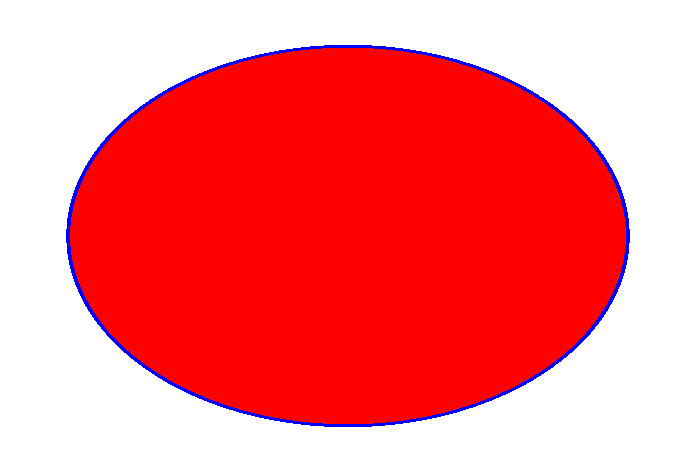# Polytest 1¶

This macro is testing the "compacting" algorithm in TPadPainter. It reduces the number of polygon's vertices using actual pixel coordinates.

It's not really useful, but just to test that the resulting polygon is still reasonable. Initial number of points is 1000000, after "compression" it's 523904 (with default canvas size, before you tried to resize it) - so almost half of vertices were removed but you can still see the reasonable shape. If you resize a canvas to a smaller size, the number of vertices after compression can be something like 5000 and even less. It's easy to 'fool' this algorithm though in this particular case (ellipse is a kind of fringe case, you can easily have a sequence of almost unique vertices (at a pixel level).

Author: Timur Pocheptsov
This notebook tutorial was automatically generated with ROOTBOOK-izer from the macro found in the ROOT repository on Sunday, September 19, 2021 at 07:43 AM.

Includes for aclic.

In :
%%cpp -d
#include <cassert>
#include <vector>

#include "TRandom.h"
#include "TCanvas.h"
#include "TError.h"
#include "Rtypes.h"
#include "TNamed.h"
#include "TMath.h"

class PolyTest1 : public TNamed, public TAttLine, public TAttFill {
public:
PolyTest1(unsigned nVertices);

void Paint(const Option_t *notUsed);
void Reset(unsigned nVertices);

private:
enum {
kNPointsDefault = 10000//minimal number of points.
};

std::vector<Double_t> fXs;
std::vector<Double_t> fYs;
};

PolyTest1::PolyTest1(unsigned nVertices)
: TNamed("polygon_compression_test1", "polygon_compression_test1")
{
Reset(nVertices);
}


In :
%%cpp -d
void PolyTest1::Reset(unsigned nVertices)
{
//Some canvas must already exist by this point.
//We need a gRandom to exist.
assert(gRandom != 0 && "Reset, gRandom is null");

if (nVertices < kNPointsDefault) {
Warning("Reset", "resetting nVertices parameter to %u", unsigned(kNPointsDefault));
nVertices = kNPointsDefault;
}

fXs.resize(nVertices);
fYs.resize(nVertices);

Double_t xMin = 0., xMax = 0., yMin = 0., yMax = 0.;
assert(xMax - xMin > 0 && yMax - yMin > 0 && "Reset, invalid canvas' ranges");

const Double_t xCentre = xMin + 0.5 * (xMax - xMin);
const Double_t yCentre = yMin + 0.5 * (yMax - yMin);

const Double_t r = TMath::Min(xMax - xMin, yMax - yMin) * 0.8 / 2;
const Double_t angle = TMath::TwoPi() / (nVertices - 1);

for (unsigned i = 0; i < nVertices - 1; ++i) {
const Double_t currR = r + gRandom->Rndm() * r * 0.01;
fXs[i] = xCentre + currR * TMath::Cos(angle * i);
fYs[i] = yCentre + currR * TMath::Sin(angle * i);
}

fXs[nVertices - 1] = fXs;
fYs[nVertices - 1] = fYs;
}


In :
%%cpp -d
void PolyTest1::Paint(const Option_t * /*notUsed*/)
{

TAttFill::Modify();

TAttLine::Modify();
}

In :
TCanvas * const cnv = new TCanvas;
cnv->cd();

PolyTest1 * polygon = new PolyTest1(1000000);
polygon->SetLineColor(kBlue);
polygon->SetFillColor(kRed);
polygon->SetLineWidth(1);
polygon->Draw();//Attach a polygon to a canvas.


Draw all canvases

In :
gROOT->GetListOfCanvases()->Draw()